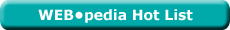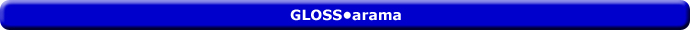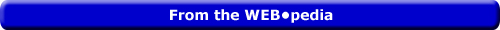Friday  May 27, 2022
 AmosWEB means Economics with a Touch of Whimsy!NUMBER OF BUYERS: One of the five demand determinants assumed constant when a demand curve is constructed, and that shift the demand curve when they change. The other four are income, preferences, other prices, and buyers' expectations. This determinant is based on the simple observation that if more people are willing and able to buy a good, then demand is greater.Most Viewed (Number) Visit the WEB*pediaARC ELASTICITY: The average elasticity for discrete changes in two variables, A and B. The distinguishing characteristic of arc elasticity is that percentage changes are calculated based on the average of the initial and ending values of each variable, rather than only initial values. Arc elasticity is generally calculated using the midpoint formula. Arc elasticity should be compared with point elasticity. For infinitesimally small changes in variables A and B, arc elasticity is the same as point elasticity.

See also | elasticity | variable | point elasticity | midpoint formula |Recommended Citation:

ARC ELASTICITY, AmosWEB GLOSS*arama, http://www.AmosWEB.com, AmosWEB LLC, 2000-2022. [Accessed: May 27, 2022].

AmosWEB Encyclonomic WEB*pedia:

Additional information on this term can be found at:

WEB*pedia: arc elasticity

Search Again?AGGREGATE EXPENDITURES EQUATION

An equation that summarizes the four aggregate expenditures on gross domestic product by the four macroeconomic sectors. In the study of Keynesian economics, this equation is commonly used to summarize the demand side of the macroeconomy. The aggregate expenditures equation actually comes in three different versions depending on how many of the four sectors and their expenditures are included.BEIGE MUNDORTLE[What's This?] Today, you are likely to spend a great deal of time watching the shopping channel trying to buy either clothing for your pet iguana or a set of hubcaps. Be on the lookout for pencil sharpeners with an attitude.Your Complete ScopeThe first paper notes printed in the United States were in denominations of 1 cent, 5 cents, 25 cents, and 50 cents."He who has a „whyľ to live can bear with almost any „how.""-- Friedrich Nietzsche, PhilosopherAOQAverage Outgoing QualityA PEDestrian's Guide Xtra CreditTell us what you think about AmosWEB. Like what you see? Have suggestions for improvements? Let us know. Click the User Feedback link.| | | | | | | | | | |
| | | |

Thanks for visiting AmosWEB